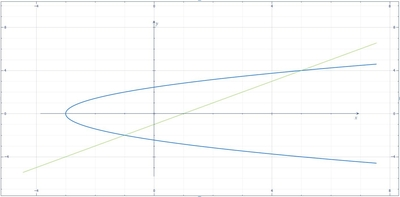# Determine the area of the region bounded by x = \frac{1}{2} y^2 - 3 and y = x - 1.

## Question:

Determine the area of the region bounded by {eq}x = \frac{1}{2} y^2 - 3 {/eq} and y = x - 1.

## Area of the Region :

One of the methods to solve the area of the region is using the formula {eq}A=\int_{a}^{b}\left [ f(y)-g(y) \right ]dy {/eq} where {eq}f(y) {/eq} and {eq}g(y) {/eq} are the two functions. The intersections between the equations are the limits of integration.

Below is the graph,The intersections between the given equations are,

{eq}\frac{1}{2}y^{2}-3=y+1 {/eq}

{eq}y^{2}-2y-8=0 {/eq}

{eq}\l...

Become a Study.com member to unlock this answer! Create your account